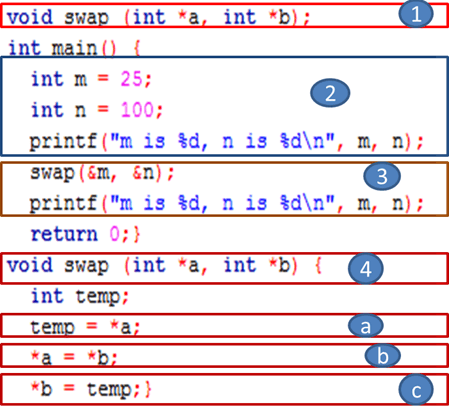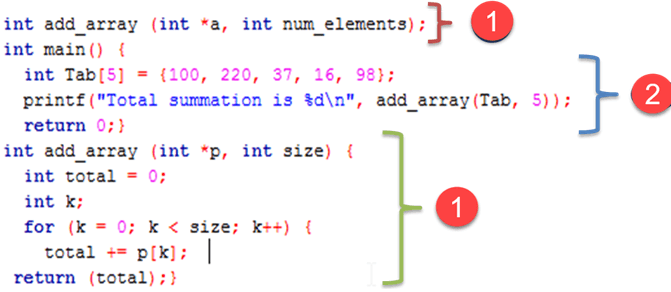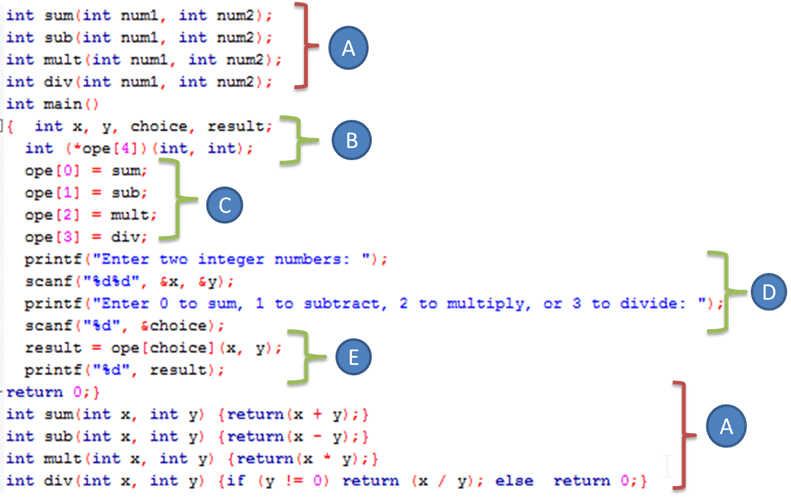# Functions Pointers in C Programming with Examples

## Functions Pointers in C Programming with Examples

Pointers give greatly possibilities to ‘C’ functions which we are limited to return one value. With pointer parameters, our functions now can process actual data rather than a copy of data.

In order to modify the actual values of variables, the calling statement passes addresses to pointer parameters in a function.

In this tutorial, you will learn-

## Functions Pointers Example

For example, the next program swaps two values of two:

```void swap (int *a, int *b);
int main() {
int m = 25;
int n = 100;
printf("m is %d, n is %d\n", m, n);
swap(&m, &n);
printf("m is %d, n is %d\n", m, n);
return 0;}
void swap (int *a, int *b) {
int temp;
temp = *a;
*a = *b;
*b = temp;}
}
```

Output:

```m is 25, n is 100
m is 100, n is 25
```The program swaps the actual variables values because the function accesses them by address using pointers. Here we will discuss the program process:

1. We declare the function responsible for swapping the two variable values, which takes two integer pointers as parameters and returns any value when it is called.
2. In the main function, we declare and initialize two integer variables (‘m’ and ‘n’) then we print their values respectively.
3. We call the swap() function by passing the address of the two variables as arguments using the ampersand symbol. After that, we print the new swapped values of variables.
4. Here we define the swap() function content which takes two integer variable addresses as parameters and declare a temporary integer variable used as a third storage box to save one of the value variables which will be put to the second variable.
5. Save the content of the first variable pointed by ‘a’ in the temporary variable.
6. Store the second variable pointed by b in the first variable pointed by a.
7. Update the second variable (pointed by b) by the value of the first variable saved in the temporary variable.

### Functions with Array Parameters

In C, we cannot pass an array by value to a function. Whereas, an array name is a pointer (address), so we just pass an array name to a function which means to pass a pointer to the array.

For example, we consider the following program:

```int add_array (int *a, int num_elements);
int main() {
int Tab = {100, 220, 37, 16, 98};
printf("Total summation is %d\n", add_array(Tab, 5));
return 0;}
int add_array (int *p, int size) {
int total = 0;
int k;
for (k = 0; k < size; k++) {
total += p[k];  /* it is equivalent to total +=*p ;p++; */}
return (total);}
```

Output:

` Total summation is 471`

Here, we will explain the program code with its details1. We declare and define add_array() function which takes an array address( pointer) with its elements number as parameters and returns the total accumulated summation of these elements. The pointer is used to iterate the array elements (using the p[k] notation), and we accumulate the summation in a local variable which will be returned after iterating the entire element array.
2. We declare and initialize an integer array with five integer elements. We print the total summation by passing the array name (which acts as address) and array size to the add_array()called function as arguments.

### Functions that Return an Array

In C, we can return a pointer to an array, as in the following program:

```#include <stdio.h>
int * build_array();
int main() {
int *a;
a = build_array(); /* get first 5 even numbers */
for (k = 0; k < 5; k++)
printf("%d\n", a[k]);
return 0;}
int * build_array() {
static int Tab={1,2,3,4,5};
return (Tab);}
```

Output:

```1
2
3
4
5
```

And here, we will discuss the program details1. We define and declare a function which returns an array address containing an integer value and didn’t take any arguments.
2. We declare an integer pointer which receives the complete array built after the function is called and we print its contents by iterating the entire five element array.

Notice that a pointer, not an array, is defined to store the array address returned by the function. Also notice that when a local variable is being returned from a function, we have to declare it as static in the function.

### Function Pointers

As we know by definition that pointers point to an address in any memory location, they can also point to at the beginning of executable code as functions in memory.

A pointer to function is declared with the * ,the general statement of its declaration is:

```return_type (*function_name)(arguments)
```

You have to remember that the parentheses around (*function_name) are important because without them, the compiler will think the function_name is returning a pointer of return_type.

After defining the function pointer, we have to assign it to a function. For example, the next program declares an ordinary function, defines a function pointer, assigns the function pointer to the ordinary function and after that calls the function through the pointer:

```#include <stdio.h>
void Hi_function (int times); /* function */
int main() {
void (*function_ptr)(int);  /* function pointer Declaration */
function_ptr = Hi_function;  /* pointer assignment */
function_ptr (3);  /* function call */
return 0;}
void Hi_function (int times) {
int k;
for (k = 0; k < times; k++) printf("Hi\n");} ```

Output:

```Hi
Hi
Hi
```1. We define and declare a standard function which prints a Hi text k times indicated by the parameter times when the function is called
2. We define a pointer function (with its special declaration) which takes an integer parameter and doesn’t return anything.
3. We initialize our pointer function with the Hi_function which means that the pointer points to the Hi_function().
4. Rather than the standard function calling by taping the function name with arguments, we call only the pointer function by passing the number 3 as arguments, and that’s it!

Keep in mind that the function name points to the beginning address of the executable code like an array name which points to its first element. Therefore, instructions like function_ptr = &Hi_function and (*funptr)(3) are correct.

NOTE: It is not important to insert the address operator & and the indirection operator * during the function assignment and function call.

### Array of Function Pointers

An array of function pointers can play a switch or an if statement role for making a decision, as in the next program:

```#include <stdio.h>
int sum(int num1, int num2);
int sub(int num1, int num2);
int mult(int num1, int num2);
int div(int num1, int num2);

int main()
{  int x, y, choice, result;
int (*ope)(int, int);
ope = sum;
ope = sub;
ope = mult;
ope = div;
printf("Enter two integer numbers: ");
scanf("%d%d", &x, &y);
printf("Enter 0 to sum, 1 to subtract, 2 to multiply, or 3 to divide: ");
scanf("%d", &choice);
result = ope[choice](x, y);
printf("%d", result);
return 0;}

int sum(int x, int y) {return(x + y);}
int sub(int x, int y) {return(x - y);}
int mult(int x, int y) {return(x * y);}
int div(int x, int y) {if (y != 0) return (x / y); else  return 0;}
```
```Enter two integer numbers: 13 48
Enter 0 to sum, 1 to subtract, 2 to multiply, or 3 to divide: 2
624
```

Here, we discuss the program details:1. We declare and define four functions which take two integer arguments and return an integer value. These functions add, subtract, multiply and divide the two arguments regarding which function is being called by the user.
2. We declare 4 integers to handle operands, operation type, and result respectively. Also, we declare an array of four function pointer. Each function pointer of array element takes two integers parameters and returns an integer value.
3. We assign and initialize each array element with the function already declared. For example, the third element which is the third function pointer will point to multiplication operation function.
4. We seek operands and type of operation from the user typed with the keyboard.
5. We called the appropriate array element (Function pointer) with arguments, and we store the result generated by the appropriate function.

The instruction int (*ope)(int, int); defines the array of function pointers. Each array element must have the same parameters and return type.

The statement result = ope[choice](x, y); runs the appropriate function according to the choice made by the user The two entered integers are the arguments passed to the function.

### Functions Using void Pointers

Void pointers are used during function declarations. We use a void * return type permits to return any type. If we assume that our parameters do not change when passing to a function, we declare it as const.

For example:

` void * cube (const void *); `

Consider the following program:

```#include <stdio.h>
void* cube (const void* num);
int main() {
int x, cube_int;
x = 4;
cube_int = cube (&x);
printf("%d cubed is %d\n", x, cube_int);
return 0;}

void* cube (const void *num) {
int result;
result = (*(int *)num) * (*(int *)num) * (*(int *)num);
return result;}
```

Result:

` 4 cubed is 64 `

Here, we will discuss the program details:1. We define and declare a function that returns an integer value and takes an address of unchangeable variable without a specific data type. We calculate the cube value of the content variable (x) pointed by the num pointer, and as it is a void pointer, we have to type cast it to an integer data type using a specific notation (* datatype) pointer, and we return the cube value.
2. We declare the operand and the result variable. Also, we initialize our operand with value “4.”
3. We call the cube function by passing the operand address, and we handle the returning value in the result variable

### Function Pointers as Arguments

Another way to exploit a function pointer by passing it as an argument to another function sometimes called “callback function” because the receiving function “calls it back.”

In the stdlib.h header file, the Quicksort “qsort()” function uses this technique which is an algorithm dedicated to sort an array.

```void qsort(void *base, size_t num, size_t width, int (*compare)(const void *, const void *))
```
• void *base : void pointer to the array.
• size_t num : The array element number.
• size_t width The element size.
• int (*compare (const void *, const void *) : function pointer composed of two arguments and returns 0 when the arguments have the same value, <0 when arg1 comes before arg2, and >0 when arg1 comes after arg2.

The following program sorts an integers array from small to big number using qsort() function:

```#include <stdio.h>
#include <stdlib.h>
int compare (const void *, const void *);
int main() {
int arr = {52, 14, 50, 48, 13};
int num, width, i;
num = sizeof(arr)/sizeof(arr);
width = sizeof(arr);
qsort((void *)arr, num, width, compare);
for (i = 0; i < 5; i++)
printf("%d ", arr[ i ]);
return 0;}

int compare (const void *elem1, const void *elem2) {
if ((*(int *)elem1) == (*(int *)elem2))  return 0;
else if ((*(int *)elem1) < (*(int *)elem2)) return -1;
else return 1;}
```

Result:

` 13 14 48 50 52 `

Here, we will discuss the program details:1. We define compare function composed of two arguments and returns 0 when the arguments have the same value, <0 when arg1 comes before arg2, and >0 when arg1 comes after arg2.The parameters are a void pointers type casted to the appropriate array data type (integer)
2. We define and initialize an integer array The array size is stored in the num variable and the size of each array element is stored in width variable using sizeof() predefined C operator.
3. We call the qsort function and pass the array name, size, width, and comparison function defined previously by the user in order to sort our array in ascending order.The comparison will be performed by taking in each iteration two array elements until the entire array will be sorted.
4. We print the array elements to be sure that our array is well sorted by iterating the entire array using for loop.